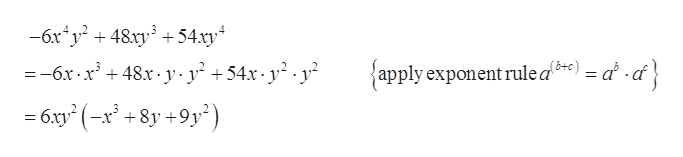How do you completely factor out on this equation ?And so what are the steps to factor out −6x4y2 + 48xy3 + 54xy4

Question

How do you completely factor out on this equation ?And so what are the steps to factor out

−6x4y2 + 48xy3 + 54xy4

Step 1

To determine the factor of given equation.

Step 2

Given equation:

Step 3

Simplify the eq...help_outlineImage Transcriptionclose6xy2 48xy354xy =-6x 48.x- y y +54x-y2.y {apply exponent rule a*) = a -cf} 6xy2 (-x +8y+9y') fullscreen

Want to see the full answer?

See Solution

Want to see this answer and more?

Our solutions are written by experts, many with advanced degrees, and available 24/7

See Solution
Tagged in

Functions